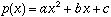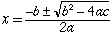My name is Sarah and I'm a Calculus student. My question is this: If f(x)=2x3+4x2-9x+8 and the derivative is f'(x)=6x2+8x-9, how do I find the critical points if f'(x) is not factorable?? Any help will be greatly appreciated Hi Sarah, If you have a quadratic you can always use the general quadratic to solve for the roots, even if you can't factor the expression. Ifthen the roots areCheers, Penny Go to Math Central